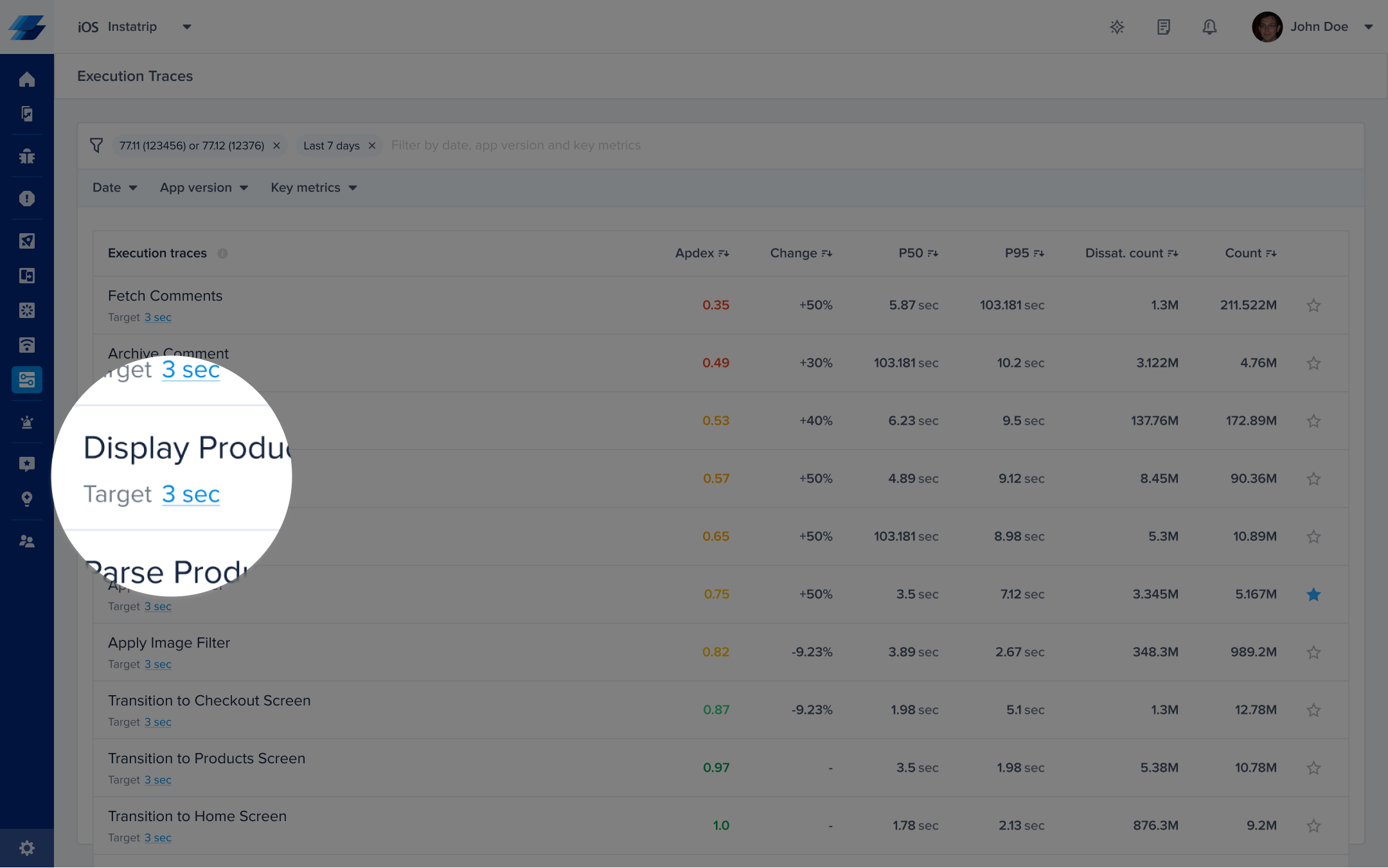# Metrics & Dimensions

The purpose of this page is to help you get started with APM for your iOS apps by learning the definition of the dimensions we currently display on metrics lists pages.

# Apdex

Apdex is an industry-standard metric used to track and measure user satisfaction based on trace latency/response times. This metric provides a standard for you to compare transactional performances, understand which ones may require additional optimization or investigation, and set targets or goals for performance. An Apdex score ranges between 0 and 1; the higher the value, the better:

• Apdex score ≥ of 0.94 equates to _Excellent _performance.
• Apdex score ≥ 0.85 and < 0.94 equates to _Good _performance.
• Apdex score ≥ 0.7 and < 0.85 equates to _Fair _performance.
• Apdex score ≥ 0.5 and < 0.7 equates to _Poor _performance.
• Apdex score < 0.5 is considered Unacceptable performance.

## How Is the App Trace Apdex Calculated?

When a trace occurrence is collected, it's flagged based on a pre-defined target (T). An app trace occurrence is considered:

• Satisfying: if its duration ≤ T
• Tolerable: if its duration > T and ≤ 4T
• Frustrating: if its duration > 4T

Then based on the bucketing explained above, the Apdex score is calculated as follows:

Total occurrences = Satisfying occurrences + Tolerable occurrences + Frustrating occurrences
Apdex score = (Satisfying occurrences + 0.5 * Tolerable occurrences) / Total occurrences

## How Can You Control a Specific Trace's Target?

By default, the target is set to 2 seconds. However, you can easily change this number from your dashboard by clicking on the action highlighted in the screenshots below.# P50

This is the 50th percentile, which is the maximum latency that 50% of all trace occurrences have in the selected time period.

# P95

This is the 95th percentile, which is the maximum latency that 95% of all trace occurrences have in the selected time period.

# Dissat. Count

The dissatisfied count is a frequency-weighted performance metric to assess the relative magnitude of your trace performance. While you can use Apdex to assess the performance of a trace compared to others, Dissat. Count takes into consideration how frequent/common this trace is and how frustrating it is to your users. This gives you one metric that you can sort your traces with to help you see the overall impact on your users.

## How Is the Dissat. Count Calculated?

This Dissat. Count is calculated with the following formula:
Dissat. Count = (1-apdex) * total occurences = total number of frustrating occurrences + 1/2 tolerable occurrences

# Count

This is the total number of occurrences in the selected time period.